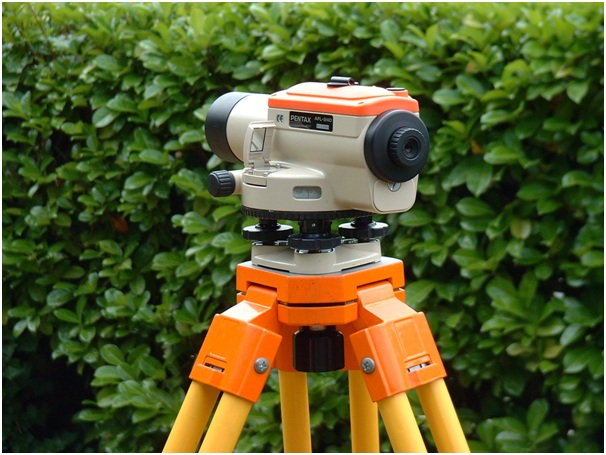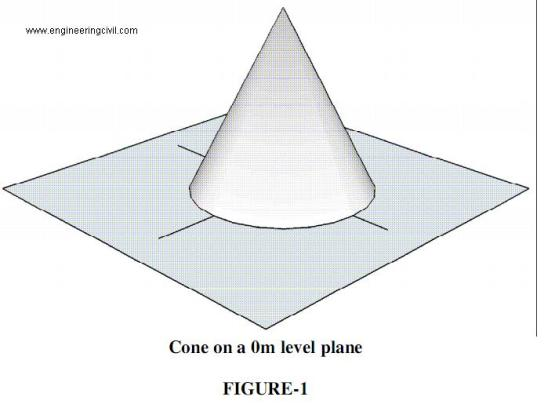Awarded as the best online publication by CIDC

# Surveying

Levelling instruments and accessories

Keywords: Levelling, dumpy level, level, levelling staff, telescope

In the previous articles we have seen that what is levelling and what are the major types of levelling employed in different circumstances. Let’s move on to the next topic in this section, which is levelling instruments and accessories.

Methods of Levelling | Classification of Direct levelling methods

Keywords: Levelling, Direct levelling, Trigonometric levelling, Barometric levelling, Hypsometric levelling, Simple levelling, Differential levelling/Compound levelling, Check levelling, Profile levelling, Cross-section levelling, Precise levelling, Reciprocal levelling

Levelling | Purpose | Terminologies

Keywords: Levelling, Surveying, Dumpy level, benchmark, Mean Sea Level, M.S.L, Elevation, G.T.S benchmarks, arbitrary benchmarks, datum, Bombay port, Reduced level, Survey of India

What is Levelling?
Levelling is defined as the process of determining the relative heights or elevations of points or objects on the earth’s surface. The elevation of a point is defined as its vertical distance measured above or below a reference level which is called datum. The most widely used datum surface is the Mean Sea Level, M.S.LLevelling instrument: Dumpy level (Source: Wikimedia Commons)

Give Introduction of Contouring

Contouring is the science of representing the vertical dimension of the terrain on a two dimensional map. We can understand contouring by considering a simple example.

Let us assume that a right circular cone of base 5m diameter and vertical height 5m is standing upright on its base. Let the base be resting on a horizontal plane at zero level as shown in Figure 1.(more…)

Explain Terms Used in Contouring

Define Contour Line
A Contour line is an imaginary outline of the terrain obtained by joining its points of equal elevation. In our example of the cone, each circle is a contour line joining points of same level.

Define Contour Interval (CI)
Contour interval is the difference between the levels of consecutive contour lines on a map. The contour interval is a constant in a given map. In our example, the contour interval is 1m.
(more…)